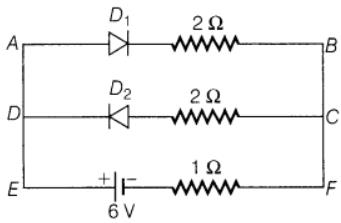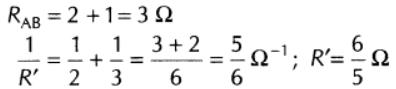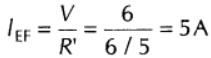Courses

# Semiconductor Diode NAT Level - 1

## 10 Questions MCQ Test Topic wise Tests for IIT JAM Physics | Semiconductor Diode NAT Level - 1

Description
This mock test of Semiconductor Diode NAT Level - 1 for IIT JAM helps you for every IIT JAM entrance exam. This contains 10 Multiple Choice Questions for IIT JAM Semiconductor Diode NAT Level - 1 (mcq) to study with solutions a complete question bank. The solved questions answers in this Semiconductor Diode NAT Level - 1 quiz give you a good mix of easy questions and tough questions. IIT JAM students definitely take this Semiconductor Diode NAT Level - 1 exercise for a better result in the exam. You can find other Semiconductor Diode NAT Level - 1 extra questions, long questions & short questions for IIT JAM on EduRev as well by searching above.
*Answer can only contain numeric values
QUESTION: 1

### A 60 V peak full wave rectified voltage is applied to a capacitor input filter. If f = 120Hz, RL=10Ω and C=10μF, the ripple voltage (V) is :

Solution:

Peak to peak ripple voltage for capacitor input filter,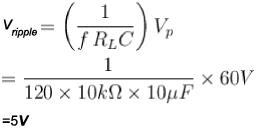*Answer can only contain numeric values
QUESTION: 2

### If a zener diode (Vz = 5V and lz = 10 mA) is connected in series with a resistance and 20V is applied across the combination, then the maximum resistance one can use without spoiling zener action (in kΩ) is :

Solution:

The principal use of a zener diode is in a voltage regulator. In a voltage regulator, the voltage across the load remains constant over a range of current.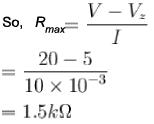*Answer can only contain numeric values
QUESTION: 3

### If the peak output voltage of a full-wave rectifier is 10V, its dc voltage (V) is :

Solution: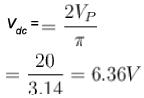*Answer can only contain numeric values
QUESTION: 4

For the rectifier circuit shown in figure, the sinusoidal voltage (V1 or V2) at the output transformer has a maximum value of 10 V. The load resistance RL is 1kΩ. What is the value of lav as a multiple of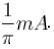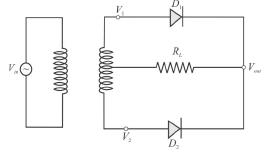Solution: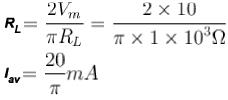*Answer can only contain numeric values
QUESTION: 5

What is the diode current (A) in the circuit: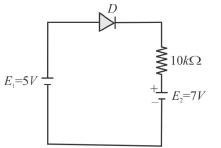Solution:

Diode will be in conducting state, when forward biased voltage is applied to it and in non-conducting state, when reverse biased voltage is applied to it. Now, the two batteries may be replaced by a single battery of 2Vwhich will make the diode reverse biased and diode goes to non-conducting state and hence current is zero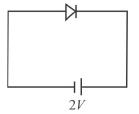*Answer can only contain numeric values
QUESTION: 6

A zener diode has a breakdown voltage of 9.1 V with maximum power dissipation of 364mW. What the maximum current A that the diode can withstand?

Solution:

Maximum current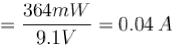*Answer can only contain numeric values
QUESTION: 7

For a zener diode, Vz = 20 V and power rating of 0.2W. If the source battery provides a potential difference of 50 V. The minimum value of Rs in Ω that prevents zener diode from being destroyed is.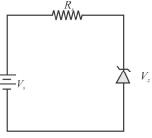Solution:

Maximum zener current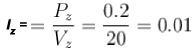Minimum value of Rs=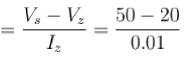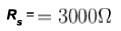*Answer can only contain numeric values
QUESTION: 8

A silicon diode is in series with a 1.0kΩ resistor and a 5V battery. If the node is connected to the positive battery terminal, the cathode voltage with respect to the negative battery terminal is (in Volts)

Solution:

The cathode voltage with respect to the negative battery terminal is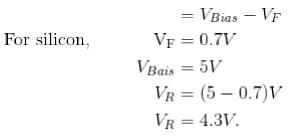*Answer can only contain numeric values
QUESTION: 9

If RL = 1kΩ, find the maximum zener current (in mA).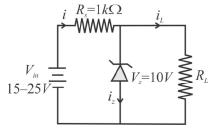Solution: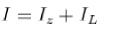Voltage developed across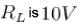always.
For maximum current l (and hence also zener current lz),Vin = 25V.
Applying KVL across loop 1, we have
(25 -10 ) = 10 x 103 x l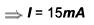Hence, maximum zener current.
lz = (15 - 10)
= 5mA

*Answer can only contain numeric values
QUESTION: 10

Assuming that the two diode D1 and D2 used in the electric circuit shown in the figure are ideal, find out the value of the current flowing through 1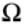resistor.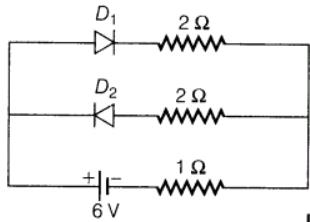Solution:

According to the question